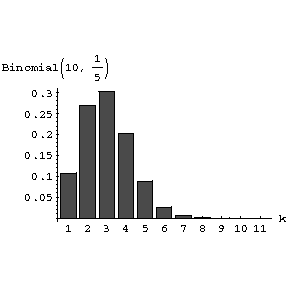# Asymmetry coefficient

The most frequently employed measure of the asymmetry of a distribution, defined by the relationship

$$\gamma _ {1} = \frac{\mu _ 3}{\mu _ {2} ^ {3/2} } ,$$

where $\mu _ {2}$ and $\mu _ {3}$ are the second and third central moments of the distribution, respectively. For distributions that are symmetric with respect to the mathematical expectation, $\gamma _ {1} = 0$; depending on the sign of $\gamma _ {1}$ one speaks of positive asymmetry ( $\gamma _ {1} > 0$) and negative asymmetry ( $\gamma _ {1} < 0$). In the case of the binomial distribution corresponding to $n$[[ Bernoulli trials|Bernoulli trials]] with probability of success $p$,

$$\tag{* } \gamma _ {1} = \frac{1 - 2 p }{\sqrt {np ( 1 - p ) }} ,$$

one has: If $p = 1/2 ( \gamma _ {1} = 0 )$, the distribution is symmetric; if $p < 1/2$ or $p > 1/2$, one obtains typical distribution diagrams with a positive (Fig.a) and negative (Fig.b) asymmetry.Figure: a013590a

$P(k, 10, 1/5 )$. Diagram of the binomial distribution $P(k, n, p)$ corresponding to $n = 10$ Bernoulli trials, with positive asymmetry ( $p = 1/5$).Figure: a013590b

$P(k, 10, 4/5 )$. Diagram of the binomial distribution $P(k, n, p)$ corresponding to $n = 10$ Bernoulli trials, with negative asymmetry ( $p = 4/5$).

The asymmetry coefficient (*) tends to zero as $n \rightarrow \infty$, in accordance with the fact that a normalized binomial distribution converges to the standard normal distribution.

The asymmetry coefficient and the excess coefficient are the most extensively used characteristics of the accuracy with which the distribution function $F _ {n} (x)$ of the normalized sum

$$\frac{( X _ {1} + \dots + X _ {n} ) - n \mu _ {1} }{\sqrt {n \mu _ {2} }} ,$$

where $X _ {1} \dots X _ {n}$ are identically distributed and mutually independent with asymmetry coefficient $\delta _ {1}$, may be approximated by the normal distribution function

$$\Phi (x) = \frac{1}{\sqrt {2 \pi }} \int\limits _ {- \infty } ^ { x } e ^ {-z ^ {2} /2 } dz .$$

Under fairly general conditions the Edgeworth series yields

$$F _ {n} (x) = \Phi (x) - \frac{1}{\sqrt n} \frac{\gamma _ 1}{6} \Phi ^ {(3)} (x) + O \left ( \frac{1}{n} \right ) ,$$

where $\Phi ^ {(3)} (x)$ is the derivative of order three.

How to Cite This Entry:
Asymmetry coefficient. Encyclopedia of Mathematics. URL: http://encyclopediaofmath.org/index.php?title=Asymmetry_coefficient&oldid=45287
This article was adapted from an original article by A.V. Prokhorov (originator), which appeared in Encyclopedia of Mathematics - ISBN 1402006098. See original article